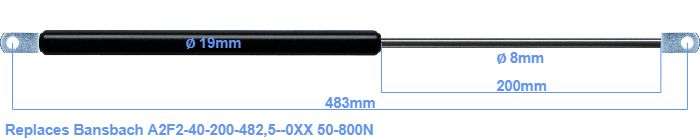# Replacement for Bansbach A2F2-40-200-482,5–0XX 50-800N

\$36.31

Replacement gas spring for the Bansbach A2F2-40-200-482,5--0XX 50-800 Newton. The eye has a thickness of 10mm and a hole diameter of 8.1mm. Brand: HAHN, a Stabilus company.

Please note:
Mounting part rod : The thickness of the eye of this replacement is 10mm instead of 18mm.
 Force Choose an option50 Newton60 Newton80 Newton100 Newton120 Newton140 Newton150 Newton160 Newton180 Newton200 Newton220 Newton240 Newton250 Newton260 Newton280 Newton300 Newton320 Newton340 Newton350 Newton360 Newton380 Newton400 Newton420 Newton440 Newton450 Newton460 Newton480 Newton500 Newton520 Newton540 Newton550 Newton560 Newton580 Newton600 Newton620 Newton640 Newton650 Newton660 Newton680 Newton700 Newton720 Newton740 Newton750 Newton760 Newton780 Newton800 NewtonClearThe diameter of the black cylinder of this gas spring is 19 millimeter. The rod has a diameter of 8 mm. The rod (the chrome part) is 200 mm long. In total, the length is 483 millimeter. Attention: this is the length between the centers of the mounting parts. Without the mounting parts this gas spring is 445 mm long (thread to thread). This replacement gas spring (A2F2-40-200-482,5--0XX) has a force of 50-800 Newton (N). This gas spring can replace a Bansbach gas spring, but is not an orignal Bansbach one. This replacement gas spring is a HAHN gas spring. Nevertheless, the dimensions and force are close to equal.